### Curve Fitting: Nonlinear Regression

### Nonlinear Regression

In nonlinear regression, the model function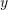is a nonlinear function ofand of the parameters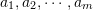. Given a set ofdata points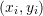with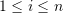, curve fitting starts by assuming a model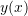withparameters. The parameters can be obtained by minimizing the least squares: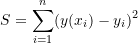In order to find the parameters of the model that would minimize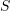,equations of the following form are solved: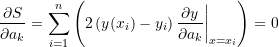Sinceis nonlinear in the coefficients, theequations formed are also nonlinear and can only be solved using a nonlinear equation solver method such as the Newton Raphson method described before. We are going to rely on the built-in NonlinearModelFit function in Mathematica that does the required calculations.

#### Example 1

The following data describes the yield strength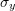and the plastic strain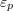obtained from uniaxial experiments where the first variable is the plastic strain and the second variable is the corresponding yield strength: (0.0001,204.18),(0.0003,226.4),(0.0015,270.35),(0.0025,276.86),(0.004,296.86),(0.005,299.3),(0.015,334.65),(0.025,346.56),(0.04,371.81),(0.05,377.45),(0.1,398.01),(0.15,422.45),(0.2,434.42).
Use the NonlinearModelFit function in Mathematica to find the best fit for the data assuming the relationship follows the form: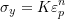##### Solution

While this model can be linearized as described in the previous section, the NonlinearModelFit will be used. The array of data is input as a list in the variable Data. The NonlinearModelFit function is then used and the output is stored in the variable: “model”. The form of the best fit function is evaluated using the built-in function Normal[model].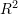can be evaluated as well as shown in the code below. The values of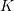andthat describe the best fit are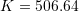and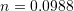. Therefore, the best-fit model is: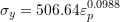The following is the Mathematica code used along with the output
View Mathematica Code
Data = {{0.0001, 204.18}, {0.0003, 226.4}, {0.0015, 270.35}, {0.0025, 276.86}, {0.004, 296.86}, {0.005, 299.3}, {0.015, 334.65}, {0.025,346.56}, {0.04, 371.81}, {0.05, 377.45}, {0.1, 398.01}, {0.15, 422.45}, {0.2, 434.42}};
model = NonlinearModelFit[Data, Kk*eps^(n), {Kk, n}, eps]
y = Normal[model]
Print["R^2=",R2 = model["RSquared"]]
Plot[y, {eps, 0, 0.21}, Epilog -> {PointSize[Large], Point[Data]}, PlotLegends -> {"Model"}, AxesLabel -> {"Eps_p", "Sigma_y"}, AxesOrigin -> {0, 0}]

View Python Code
import numpy as np
import matplotlib.pyplot as plt
from scipy.optimize import curve_fit

Data = [[0.0001, 204.18], [0.0003, 226.4], [0.0015, 270.35], [0.0025, 276.86], [0.004, 296.86], [0.005, 299.3], [0.015, 334.65], [0.025,346.56], [0.04, 371.81], [0.05, 377.45], [0.1, 398.01], [0.15, 422.45], [0.2, 434.42]]
def f(eps, k, n): return k*eps**n
coeff, covariance = curve_fit(f, [point for point in Data],
[point for point in Data])
print("coeff: ",coeff)
x_val = np.arange(0,0.21,0.001)
plt.title('%.5feps**(%.5f)' % tuple(coeff))
plt.plot(x_val, f(x_val, coeff, coeff))
plt.scatter([point for point in Data], [point for point in Data], c='k')
plt.xlabel("Eps_p"); plt.ylabel("Sigma_y")
plt.grid(); plt.show()

# R squared
x = np.array([point for point in Data])
y = np.array([point for point in Data])
y_fit = f(x, coeff, coeff)
ss_res = np.sum((y - y_fit)**2)
ss_tot = np.sum((y - np.mean(y))**2)
r2 = 1 - (ss_res / ss_tot)
print("R Squared: ",r2)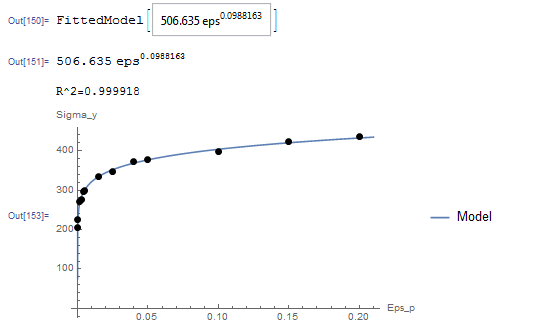The following links provide the MATLAB codes for implementing a nonlinear model fit for yield strength vs plastic strain. The function for the model is provided in File 2.

#### Example 2

Exponential and Logarithmic Models offer a wide range of model functions that can be used for various engineering applications. An example is the exponential model that describes an initial rapid increase in the dependent variablewhich then levels off to become asymptotic to an upper limit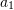: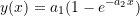whereand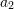are the model parameters. For plastic materials, Armstrong and Frederick proposed this model in 1966 (republished here in 2007) to describe how a quantity called the “Centre of the Yield Surface” changes with the increase in the plastic strain. Ifis the centre of the yield surface andis the plastic strain, then, the model forhas the form: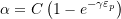Find the values of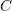and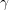for the best fit to the experimental data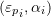given by: (0.0001,2.067),(0.005,3.96),(0.0075,6.22),(0.008,8.07),(0.009,9.32),(0.01,12.02),(0.02,13.2),(0.03,15.22),(0.04,15.51),(0.05,15.44),(0.06,15.1),(0.075,15.76),(0.1,15.11),(0.17,15.53),(0.2,15.26)

##### Solution

Unlike the model in the previous question, this model cannot be linearized. The NonlinearModelFit built-in function in Mathematica will be used. The array of data is input as a list in the variable Data. The NonlinearModelFit function is then used and the output is stored in the variable: “model”. The form of the best fit function is evaluated using the built-in function Normal[model].can be evaluated as well as shown in the code below. The values ofandthat describe the best fit are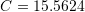and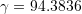. Therefore, the best-fit model is: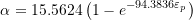The following is the Mathematica code used along with the output

View Mathematica Code
Data = {{0.0001, 2.067}, {0.005, 3.96}, {0.0075, 6.22}, {0.008,8.07}, {0.009, 9.32}, {0.01, 12.02}, {0.02, 13.2}, {0.03,15.22}, {0.04, 15.51}, {0.05, 15.44}, {0.06, 15.1}, {0.075,15.76}, {0.1, 15.11}, {0.17, 15.53}, {0.2, 15.26}};
model = NonlinearModelFit[Data, Cc (1 - E^(-gamma*eps)), {Cc, gamma}, eps]
y = Normal[model]
Print["R^2=", R2 = model["RSquared"]]
Plot[y, {eps, 0, 0.21}, Epilog -> {PointSize[Large], Point[Data]}, PlotLegends -> {"Model"}, AxesLabel -> {"Eps_p", "Alpha"}, AxesOrigin -> {0, 0}]

View Python Code
import numpy as np
import matplotlib.pyplot as plt
from scipy.optimize import curve_fit

Data = [[0.0001, 2.067], [0.005, 3.96], [0.0075, 6.22], [0.008,8.07], [0.009, 9.32], [0.01, 12.02], [0.02, 13.2], [0.03,15.22], [0.04, 15.51], [0.05, 15.44], [0.06, 15.1], [0.075,15.76], [0.1, 15.11], [0.17, 15.53], [0.2, 15.26]]
def f(eps, c, gamma): return c*(1 - np.exp(-gamma*eps))
coeff, covariance = curve_fit(f, [point for point in Data],
[point for point in Data])
print("coeff: ",coeff)
x_val = np.arange(0,0.21,0.001)
plt.title('%.5f(1 - e**(-%.5feps))' % tuple(coeff))
plt.plot(x_val, f(x_val, coeff, coeff))
plt.scatter([point for point in Data], [point for point in Data], c='k')
plt.xlabel("Eps_p"); plt.ylabel("Alpha")
plt.grid(); plt.show()

# R squared
x = np.array([point for point in Data])
y = np.array([point for point in Data])
y_fit = f(x, coeff, coeff)
ss_res = np.sum((y - y_fit)**2)
ss_tot = np.sum((y - np.mean(y))**2)
r2 = 1 - (ss_res / ss_tot)
print("R Squared: ",r2)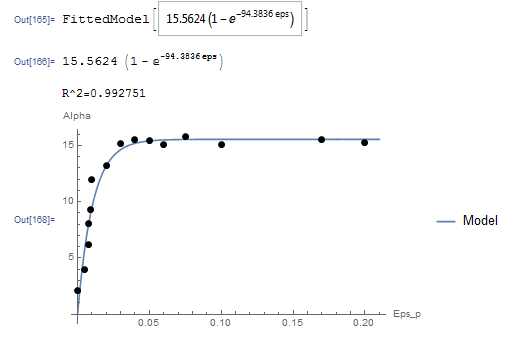The following links provide the MATLAB codes for implementing a Nonlinear model fit for centre of the yield surface. The function for the model is provided in File 2.